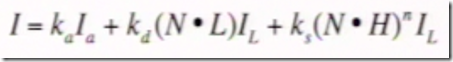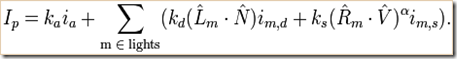Notes on lecture, 2. Illumination. What is light? The BRDF. Vectors and dot products. The Phong BRDF model (2/7) … Light-Material interactions, what is light, illumination & reflectance, phong reflectance model, vectors & dot products. … more details about vectors & dot product in lecture 3

Diffuse reflections (incident ray reflects at many angles).  Specular reflections (mirror-like, incident ray reflects at many angles).  Specular highlights (bright spot on shiny object).  Refraction (bends light).  Dispersion (e.g. rainbow) (phase velocity of wave depends on its frequency) (different refractive index for different wavelengths).  Rainbow photo shows refraction, internal reflection, dispersion.  Atmospheric effects (haze, fog, crepuscular rays / god rays, light under water).  Interference (e.g. soap bubbles, thin layer of oil on water) (two waves superimpose to form a resultant wave of greater or lower amplitude).  Diffraction (e.g. CD discs) (physically similar to interference, but diffraction tends to mean lots of interference) (wave encounters obstacle) (disc example, tiny grooves are close to the wavelength of light so it causes some of the light to reflect in phase and some out of phase).  Motion blur.

What is light, wave-particle duality, but basic CG does geometric view of optics (rays of photos), and we simulate wave behaviors as effects.  Newton dispersion.

Human eye detects light (biology).  Visible spectrum of electromagnetic waves is about 390 to 700nm.  Human eye photoreceptor cells: rods adapt to brightness (helps vision in low light), cones detect red & green & blue (trichromatic vision) (cone peaks are short 420-440 nm, middle 530-540 nm, long 560-580 nm).  Metamers (different combinations of light across all wavelengths can produce the same visible color in terms of human eye receptor response).  Humans see light as additive colors (R+G=Yellow, R+B=Magenta, G+B=Cyan, R+G+B=White).  Ink (CMYK printing) & paint & crayons uses subtractive colors (Magenta+Yellow=R, Cyan+Yellow=G, Cyan+Magenta=B, Magenta+Yellow+Cyan=Black).

Illumination light sources: emittance spectrum (color), geometry (position & direction), directional attenuation (falloff).  Illumination surface properties: reflectance spectrum (color), geometry (position, orientation, micro-structure), absorption.

Graphics jargon (he’s using): illumination is transport of energy from light sources between points (direct & indirect paths).  Lighting is compute light intensity reflected from a specific 3D point.  Shading is assign color to a pixel based on illumination in the scene.

Direct illumination: surface point receives light directly from all light sources in scene – determine which light sources are visible, compute by local illumination model; OpenGL.  Global illumination: surface point receives light after light rays interact with other objects; indirect illumination; slow, ray tracing.

Light sources.  Directional light source.  Point light source.  Other: spotlights, area light sources, extended light sources (spherical).

Light is linear – you can add light sources and components.

Reflectance models.  BRDF (bi-directional reflection distribution function).  Can measure this experimentally (with real object, light camera) for lots of angles.  Or approximate with code – physically-based models (laws of physics), or empirical models (ad hoc), or data driven (lots of data).

Phong reflectance model uses diffuse + specular + ambient.
* Diffuse Reflectance uses Lambert’s Cosine Law, dL = dA*cos(theta).  Use three vectors from object point to: viewer, normal, light.  For Diffuse, viewer vector/angle doesn’t matter; all we use is: Id = kd*(N dot L)*Il … Illumination Diffuse = Diffuse Reflection Coefficient * (Normal Vector dot Light Direction Vector) * Illumination Light Source.  Vectors must be normalized.
* Specular Reflectance is affected by view vector (object point to camera).  Special case of Snell’s Law.  Incoming light direction is same as outgoing, meaning angle between light vector & normal vector is the same as the angle between reflect vector & normal vector (angle between L & N is same as angle between R & N).  Is = ks*(V dot R)*Il => Illumination Specular = Constant Specular * (View Vector dot Reflect Vector) * Illumination Light Source.
* Non-Ideal Specular Reflectance adds an exponent to the equation: Illumination Specular = Constant Specular * (View Vector dot Reflect Vector)^Exponent * Illumination Light Source
* Blinn & Torrance Variation: Illumination Specular = Constant Specular * (View Vector dot H)^Exponent * Illumination Light Source … H vector is halfway between L (light vector) and V (view vector) => don’t need to compute reflection vector R at every point.  Also, if V is very far away and L is very far away, then Illumination Specular is just a function of N (Normal Vector)

Single light source Phong Reflectance model is Ambient + Diffuse + Specular:Equation from wikipedia for multiple light sources: